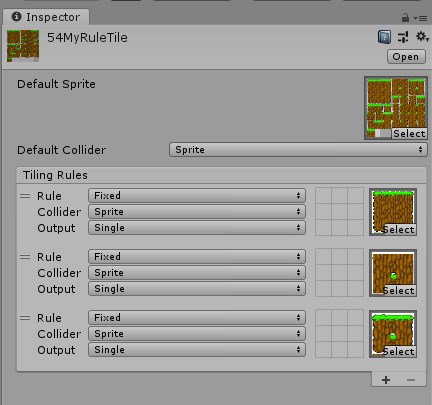# How do I add neighbor rules for RuleTiles by script?

Not much hope for this but here we go.

So far I managed to successfully create a rule tile by script, add rules to it by script, and set the sprite for each of those rules also by script.

Here’s the test code i’m making. It has a default sprite and 3 rules, each with its own sprite.

``````public static Sprite spriteDefault;
public static Sprite sprite1;
public static Sprite sprite2;
public static Sprite sprite3;

static void CreateRuleTile()
{
RuleTile ruleTile = ScriptableObject.CreateInstance("RuleTile") as RuleTile;

AssetDatabase.CreateAsset(ruleTile, "Assets/" + UnityEngine.Random.Range(0, 999).ToString() + "MyRuleTile.asset");

Debug.Log(AssetDatabase.GetAssetPath(ruleTile));
ruleTile.m_DefaultSprite = spriteDefault;

RuleTile.TilingRule rule01 = new RuleTile.TilingRule();
rule01.m_Sprites = sprite1;

RuleTile.TilingRule rule02 = new RuleTile.TilingRule();
rule02.m_Sprites = sprite2;

RuleTile.TilingRule rule03 = new RuleTile.TilingRule();
rule03.m_Sprites = sprite3;

}
``````

This create a nice little rule tile for me:Now i’m trying to add the neighbor rules (the 8 little arrows we can set manually on the editor) by script but I have no idea how to do it.

Each rule has a property called m_Neighbors and when I hover over it it says RuleTile.TilingRule.Neighbor RuleTile.TilingRule.m_Neighbors

How can I create custom neighbor rules to add to each of my rules?

After some searching in the code i found that neighbors can be set using 2 functions in the TilingRule class. This little snippet will just set all the neighbors to one single type.

``````    Dictionary<Vector3Int, int> dict = rule01.GetNeighbors();
List<Vector3Int> neighbors = rule01.m_NeighborPositions;
for(int i = 0; i < neighbors.Count; i++)
{
``````

}
rule01.ApplyNeighbors(dict);
And this is the class that can be found in RuleTile.cs:
[Serializable]
public class TilingRule : TilingRuleOutput
{
///

/// The matching Rule conditions for each of its neighboring Tiles.
///

public List m_Neighbors = new List();
///
/// * Preset this list to RuleTile backward compatible, but not support for HexagonalRuleTile backward compatible.
///

public List m_NeighborPositions = new List()
{
new Vector3Int(-1, 1, 0),
new Vector3Int(0, 1, 0),
new Vector3Int(1, 1, 0),
new Vector3Int(-1, 0, 0),
new Vector3Int(1, 0, 0),
new Vector3Int(-1, -1, 0),
new Vector3Int(0, -1, 0),
new Vector3Int(1, -1, 0),
};
///
/// The transform matching Rule for this Rule.
///

public Transform m_RuleTransform;

public Dictionary<Vector3Int, int> GetNeighbors()
{
Dictionary<Vector3Int, int> dict = new Dictionary<Vector3Int, int>();

for (int i = 0; i < m_Neighbors.Count && i < m_NeighborPositions.Count; i++)

return dict;
}

public void ApplyNeighbors(Dictionary<Vector3Int, int> dict)
{
m_NeighborPositions = dict.Keys.ToList();
m_Neighbors = dict.Values.ToList();
}

public BoundsInt GetBounds()
{
BoundsInt bounds = new BoundsInt(Vector3Int.zero, Vector3Int.one);
foreach (var neighbor in GetNeighbors())
{
bounds.xMin = Mathf.Min(bounds.xMin, neighbor.Key.x);
bounds.yMin = Mathf.Min(bounds.yMin, neighbor.Key.y);
bounds.xMax = Mathf.Max(bounds.xMax, neighbor.Key.x + 1);
bounds.yMax = Mathf.Max(bounds.yMax, neighbor.Key.y + 1);
}
return bounds;
}
}